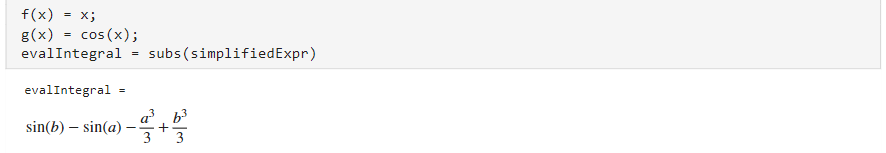# Simplify Symbolic Expressions Using Live Editor Task

Starting in R2020a, you can interactively simplify or rearrange symbolic expressions using the Simplify Symbolic Expression task in the Live Editor. For more information on Live Editor tasks, see Add Interactive Tasks to a Live Script.

This example shows you how to simplify or rearrange various symbolic expressions into the particular form you require by choosing the appropriate method.

### Simplify a Symbolic Expression

Simplify the expression $i\frac{{e}^{-ix}-{e}^{ix}}{{e}^{-ix}+{e}^{ix}}$.

First, go to the Home tab, and create a live script by clicking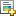New Live Script. Define the symbolic variable `x` and declare the expression as a symbolic expression.

```syms x; expr = 1i*(exp(-1i*x) - exp(1i*x))/(exp(-1i*x) + exp(1i*x));```

In the Live Editor tab, run the code by clickingRun to store `x` and `expr` into the current workspace.

Next, open the Simplify Symbolic Expression task by selecting Task > Simplify Symbolic Expression in the Live Editor tab. Select the symbolic expression `expr` from the workspace and specify the simplification method as `Simplify`. Select `Minimum` for the computational effort (fastest computation time).To get a simpler expression, change the computational effort to `Medium`.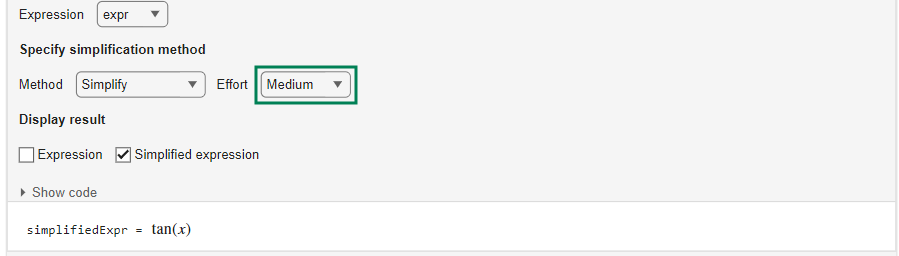To experiment with simplifying symbolic expressions, you can repeat the previous steps for other symbolic expressions and simplification methods. You can run the following examples by adding the code to the existing live script or a new live script.

### Simplify a Polynomial Fraction

Simplify the polynomial fraction $\frac{\left({x}^{2}-1\right)\left(x+1\right)}{{x}^{2}-2x+1}$.

Declare the polynomial fraction as a symbolic expression.

`expr2 = ((x^2 - 1)*(x + 1))/(x^2 - 2*x + 1);`

Select the symbolic expression `expr2` from the workspace and specify the simplification method as `Simplify fraction`.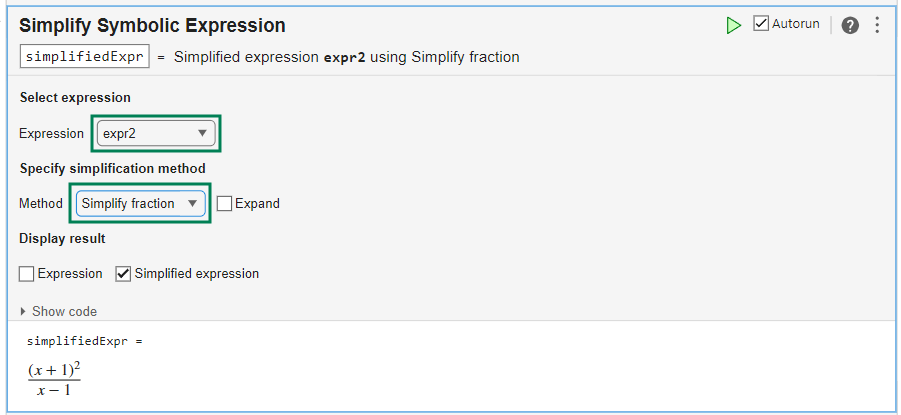Select the Expand option to return the numerator and denominator of the simplified fraction in expanded form.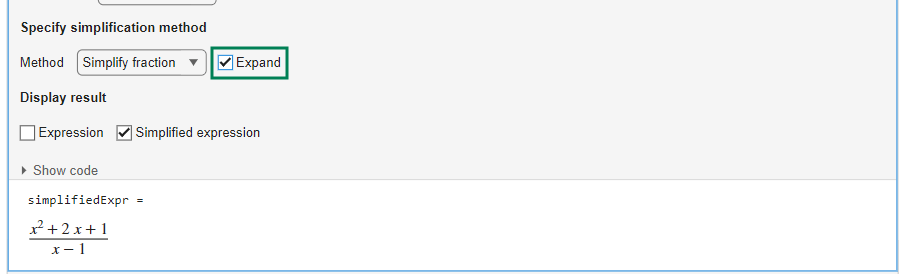### Rewrite an Expression in a Different Form

Rewrite the trigonometric function $\mathrm{tan}\left(x\right)$ in terms of the sine function.

Declare $\mathrm{tan}\left(x\right)$ as a symbolic expression.

`expr3 = tan(x);`

Select the symbolic expression `expr3` from the workspace and specify the simplification method as `Rewrite`. Choose `sin` to rewrite $\mathrm{tan}\left(x\right)$ in terms of the sine function.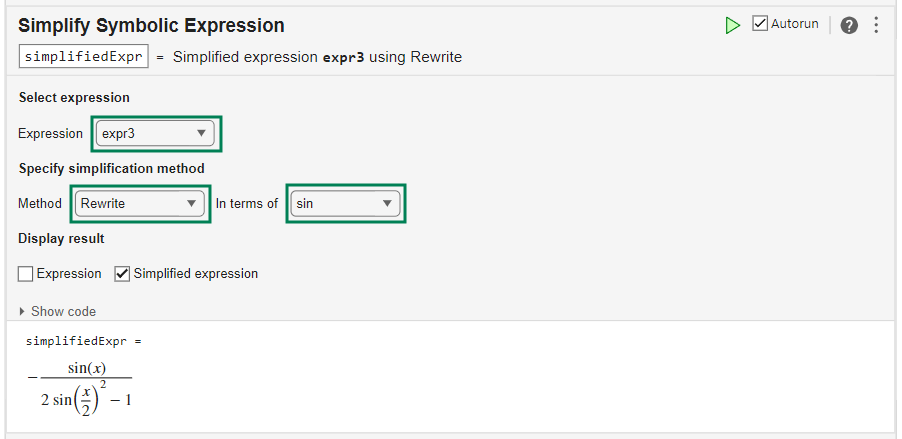### Expand a Logarithmic Expression

Expand the expression $log\left(\frac{{x}^{3}\phantom{\rule{0.16666666666666666em}{0ex}}{e}^{x}}{2}\right)$ using the logarithmic identities.

Declare the logarithmic expression as a symbolic expression.

`expr4 = log(x^3*exp(x)/2);`

Select the symbolic expression `expr4` from the workspace and specify the simplification method as `Expand`. By default, the symbolic variable `x` in `expr4` is complex when it is initially created. The `Expand` method does not simplify the input expression because the logarithmic identities are not valid for complex values of variables. To apply identities that are convenient but do not always hold for all values of variables, select the Ignore analytic constraints option.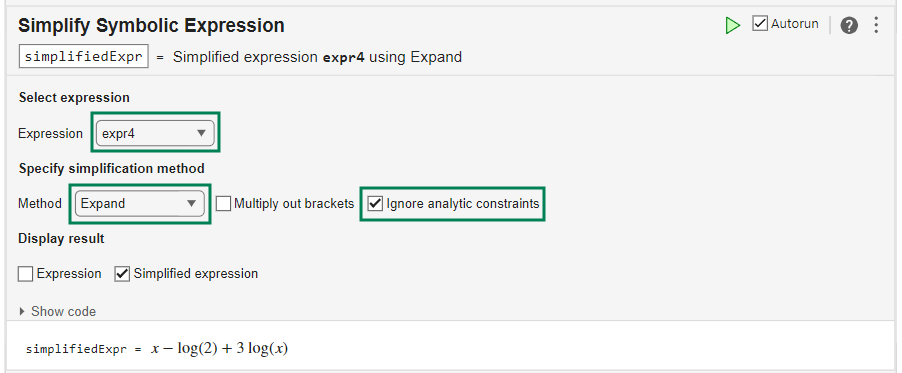### Simplify the Sum of Two Integral Expressions

Simplify the sum of two integral expressions: ${\int }_{a}^{b}x\phantom{\rule{0.16666666666666666em}{0ex}}f\left(x\right)\text{d}x+{\int }_{a}^{b}g\left(y\right)\text{d}y$.

First, define $a$ and $b$ as symbolic variables, and $f\left(x\right)$ and $g\left(y\right)$ as symbolic functions. Use the `int` function to represent the integrals.

```syms a b f(x) g(y) expr5 = int(x*f(x),x,a,b) + int(g(y),y,a,b);```

Select the symbolic expression `expr5` from the workspace and specify the simplification method as `Combine`. Choose `int` as the function to combine.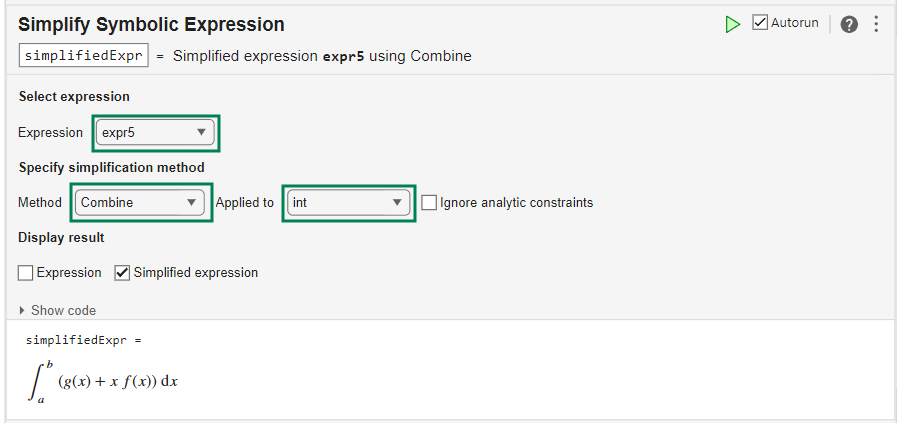### Generate Code

To view the code that a task used, click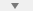at the bottom of the task window. The task displays the code block, which you can cut and paste to use or modify later in the existing script or a different program. For example: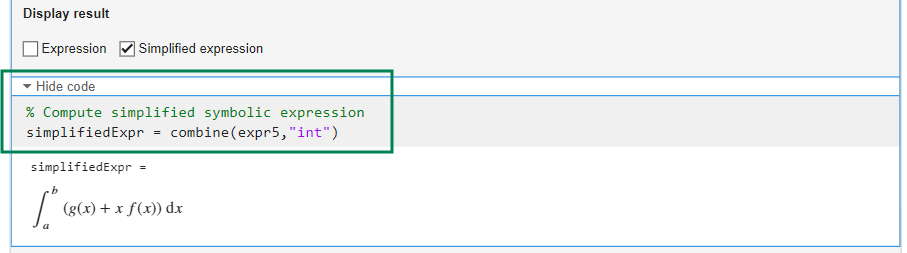Because the underlying code is now part of your live script, you can continue to use the variables created by the task for further processing. For example, define the functions $f\left(x\right)$ and $g\left(x\right)$ as $f\left(x\right)=x$ and $g\left(x\right)=\mathrm{cos}\left(x\right)$. Evaluate the integrals in `simplifiedExpr3` by substituting these functions.